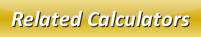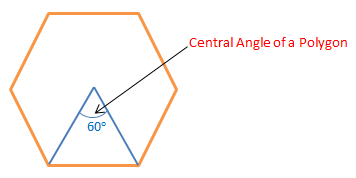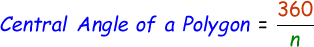# Central Angle of a Polygon Calculator

The central angle of a polygon is the angle that is subtended from one side of the polygon to its center. In other words, it is the angle which gets created when we join two adjacent vertices of the given polygon as shown in the below figure.Formula to calculate the central angle of a polygon is given by:where,
n = Number of sides of the polygon

In the below online central angle of a polygon calculator, enter the number of side of the polygon and then click calculate to find the answer.

 Number of Sides of Polygon: Central Angle of Polygon: [Degrees]

Latest Calculator Release

Average Acceleration Calculator

Average acceleration is the object's change in speed for a specific given time period. ...

Free Fall Calculator

When an object falls into the ground due to planet's own gravitational force is known a...

Torque Calculator

Torque is nothing but a rotational force. In other words, the amount of force applied t...

Average Force Calculator

Average force can be explained as the amount of force exerted by the body moving at giv...

Angular Displacement Calculator

Angular displacement is the angle at which an object moves on a circular path. It is de...